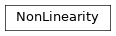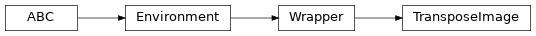# garage.torch._functions¶

Utility functions for PyTorch algorithms.

A collection of common functions that are used by Pytorch algos.

This collection of functions can be used to manage the following:
• Pytorch GPU usage
• setting the default Pytorch GPU

• converting Tensors to GPU Tensors

• Converting Tensors into numpy.ndarray format and vice versa

• Updating model parameters

compute_advantages(discount, gae_lambda, max_episode_length, baselines, rewards)

Advantages are a discounted cumulative sum.

The discounted cumulative sum can be computed using conv2d with filter. filter:

[1, (discount * gae_lambda), (discount * gae_lambda) ^ 2, …] where the length is same with max_episode_length.

baselines and rewards are also has same shape.

baselines: [ [b_11, b_12, b_13, … b_1n],

[b_21, b_22, b_23, … b_2n], … [b_m1, b_m2, b_m3, … b_mn] ]

rewards: [ [r_11, r_12, r_13, … r_1n],

[r_21, r_22, r_23, … r_2n], … [r_m1, r_m2, r_m3, … r_mn] ]

Parameters
• discount (float) – RL discount factor (i.e. gamma).

• gae_lambda (float) – Lambda, as used for Generalized Advantage Estimation (GAE).

• max_episode_length (int) – Maximum length of a single episode.

• baselines (torch.Tensor) – A 2D vector of value function estimates with shape (N, T), where N is the batch dimension (number of episodes) and T is the maximum episode length experienced by the agent. If an episode terminates in fewer than T time steps, the remaining elements in that episode should be set to 0.

• rewards (torch.Tensor) – A 2D vector of per-step rewards with shape (N, T), where N is the batch dimension (number of episodes) and T is the maximum episode length experienced by the agent. If an episode terminates in fewer than T time steps, the remaining elements in that episode should be set to 0.

Returns

A 2D vector of calculated advantage values with shape

(N, T), where N is the batch dimension (number of episodes) and T is the maximum episode length experienced by the agent. If an episode terminates in fewer than T time steps, the remaining values in that episode should be set to 0.

Return type

torch.Tensor

pad_to_last(nums, total_length, axis=- 1, val=0)

Pad val to last in nums in given axis.

length of the result in given axis should be total_length.

Raises

IndexError – If the input axis value is out of range of the nums array

Parameters
• nums (numpy.ndarray) – The array to pad.

• total_length (int) – The final width of the Array.

• axis (int) – Axis along which a sum is performed.

• val (int) – The value to set the padded value.

Returns

Return type

torch.Tensor

filter_valids(tensor, valids)

Filter out tensor using valids (last index of valid tensors).

valids contains last indices of each rows.

Parameters
• tensor (torch.Tensor) – The tensor to filter

• valids (list[int]) – Array of length of the valid values

Returns

Filtered Tensor

Return type

torch.Tensor

np_to_torch(array)

Numpy arrays to PyTorch tensors.

Parameters

array (np.ndarray) – Data in numpy array.

Returns

float tensor on the global device.

Return type

torch.Tensor

dict_np_to_torch(array_dict)

Convert a dict whose values are numpy arrays to PyTorch tensors.

Modifies array_dict in place.

Parameters

array_dict (dict) – Dictionary of data in numpy arrays

Returns

Dictionary of data in PyTorch tensors

Return type

dict

torch_to_np(tensors)

Convert PyTorch tensors to numpy arrays.

Parameters

tensors (tuple) – Tuple of data in PyTorch tensors.

Returns

Tuple of data in numpy arrays.

Return type

tuple[numpy.ndarray]

Note: This method is deprecated and now replaced by

garage.torch._functions.to_numpy.

flatten_batch(tensor)

Flatten a batch of observations.

Reshape a tensor of size (X, Y, Z) into (X*Y, Z)

Parameters

tensor (torch.Tensor) – Tensor to flatten.

Returns

Flattened tensor.

Return type

torch.Tensor

flatten_to_single_vector(tensor)

Collapse the C x H x W values per representation into a single long vector.

Reshape a tensor of size (N, C, H, W) into (N, C * H * W).

Parameters

tensor (torch.tensor) – batch of data.

Returns

Reshaped view of that data (analogous to numpy.reshape)

Return type

torch.Tensor

update_module_params(module, new_params)

This function acts like torch.nn.Module._load_from_state_dict(), but it replaces the tensors in module with those in new_params, while _load_from_state_dict() loads only the value. Use this function so that the grad and grad_fn of new_params can be restored

Parameters
• module (torch.nn.Module) – A torch module.

• new_params (dict) – A dict of torch tensor used as the new parameters of this module. This parameters dict should be generated by torch.nn.Module.named_parameters()

soft_update_model(target_model, source_model, tau)

Update model parameter of target and source model.

# noqa: D417 :param target_model:

(garage.torch.Policy/garage.torch.QFunction):

Target model to update.

Parameters
• source_model

(garage.torch.Policy/QFunction):

Source network to update.

• tau (float) – Interpolation parameter for doing the soft target update.

set_gpu_mode(mode, gpu_id=0)

Set GPU mode and device ID.

Parameters
• mode (bool) – Whether or not to use GPU

• gpu_id (int) – GPU ID

prefer_gpu()

Prefer to use GPU(s) if GPU(s) is detected.

global_device()

Returns the global device that torch.Tensors should be placed on.

Note: The global device is set by using the function

garage.torch._functions.set_gpu_mode. If this functions is never called garage.torch._functions.device() returns None.

Returns

The global device that newly created torch.Tensors

should be placed on.

Return type

torch.Device

product_of_gaussians(mus, sigmas_squared)

Compute mu, sigma of product of gaussians.

Parameters
• mus (torch.Tensor) – Means, with shape $$(N, M)$$. M is the number of mean values.

• sigmas_squared (torch.Tensor) – Variances, with shape $$(N, V)$$. V is the number of variance values.

Returns

Mu of product of gaussians, with shape $$(N, 1)$$. torch.Tensor: Sigma of product of gaussians, with shape $$(N, 1)$$.

Return type

torch.Tensor

class NonLinearity(non_linear)

Bases: torch.nn.ModuleWrapper class for non linear function or module.

Parameters

non_linear (callable or type) – Non-linear function or type to be wrapped.

forward(self, input_value)

Forward method.

Parameters

input_value (torch.Tensor) – Input values

Returns

Output value

Return type

torch.Tensor

class TransposeImage(env)

Bases: garage.WrapperTranspose observation space for image observation in PyTorch.

Reshape the input observation shape from (H, W, C) into (C, H, W)

in pytorch format.

property observation_space(self)

akro.Space: The observation space specification.

property spec(self)

EnvSpec: The environment specification.

step(self, action)

Step the wrapped env.

Parameters

action (np.ndarray) – An action provided by the agent.

Returns

The environment step resulting from the action.

Return type

EnvStep

property action_space(self)

akro.Space: The action space specification.

property render_modes(self)

list: A list of string representing the supported render modes.

reset(self)

Reset the wrapped env.

Returns

The first observation conforming to

observation_space.

dict: The episode-level information.

Note that this is not part of env_info provided in step(). It contains information of he entire episode， which could be needed to determine the first action (e.g. in the case of goal-conditioned or MTRL.)

Return type

numpy.ndarray

render(self, mode)

Render the wrapped environment.

Parameters

mode (str) – the mode to render with. The string must be present in self.render_modes.

Returns

the return value for render, depending on each env.

Return type

object

visualize(self)

Creates a visualization of the wrapped environment.

close(self)

Close the wrapped env.

property unwrapped(self)

garage.Environment: The inner environment.